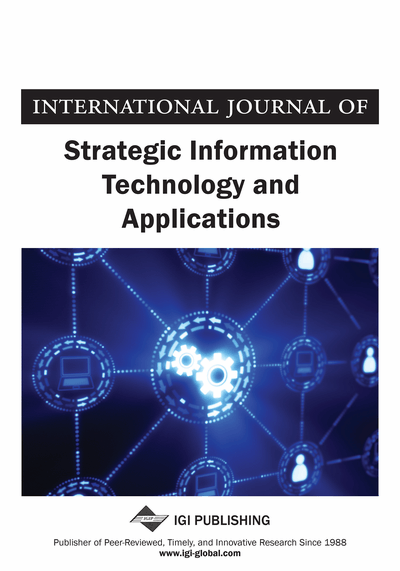# A Nearly One-to-One Method to Convert Analog Signals into a Small Volume of Data: First Part: 1-D Signals

Dan Ciulin (E-I-A Lausanne, Switzerland)
DOI: 10.4018/jsita.2010100105
Available
\$37.50
No Current Special Offers

## Abstract

The actual quantizing and sampling procedure leads to irreducible errors that do not permit to reconstruct correctly the initial analog signal. To diminish the reconstruction errors to an acceptable level one has to increase the volume of transmitted data. Then, it results in big (or even very big) data volumes that are difficult to store and/or transmit. A deal has been made sometimes between the quality and the resulted data volume in order to have small enough volumes. The paper presents a new (theoretically) one-to-one method to convert physical realizable analog signals into a small volume of data. This method uses the (Shannon) Sampling Theorem, the Bandwidth Compression Theorem and a new Quantizing and Sampling Theorem described in this paper. The Quantizing and Sampling Theorem is based on the properties of phase/frequency modulation and on the properties of a Phase Lock Loop (PLL) to decode also amplitude-modulated signals. Practically, this new method may realize a (theoretically) nearly one-to-one conversion of a physically realizable analog signal into a small volume of data. Adequate software and hardware have to be realized to achieve technically this goal. To diminish the errors of calculus, error- free calculus methods have to be used.
Article Preview
Top

## Introduction

The signals existing in nature are analogue but actual signal processing and transmission techniques are based mainly on digital techniques. Unfortunately, the actual quantizing and sampling procedure leads to irreducible errors that do not permit to reconstruct correctly the initial analog signal (Analog Devices Inc., 1974). To diminish these errors to an acceptable level, one has to increase the volume of transmitted data. Then, even for the best actual technical processing, it results volume and data quality that cannot be compared with their equivalent realized by a human brain.

It can be remarked that for both technical and/or human cases, the ‘sensed’ signal represents only a part of the physical reality but this part seems to be enough for the user in most of the cases. Anyhow, any physical realizable signal must have finite time and frequency support and also finite energy. This last constrains lead practically to finite amplitude for the signal. One can then suppose an actual upper limit for a given 1-D signal, limit that leads to nearly undiscerning errors for a human user. As example, for analog audio signals, the frequency bandwidth must be of about 16 Hz to 20 kHz or more, the signal to noise ratio more than about 140 dB in accordance of a the number of quanta of about 24 bits and its maximal amplitude depending on the used channel of transmission.

It can be also remarked that if a given coding procedure is (theoretically) ‘one-to-one’, its ‘code’ represents exactly the same signal. Then, any physical realizable signal may be considered as a ‘code’ and that explains also the ability to conceive virtually a work in our mind before we will do it in reality. A signal must be aleatory to contain information. Then, it must be ‘time variant’. For a small enough time interval, one may consider a signal as ‘stationary’ and then, represents it locally by a Fourier series. This is equivalent to say that for this time interval the signal may be considered ‘periodic’. For an even smaller time interval, the signal may be considered as having ’constant amplitude’. This is the case for the Shannon sampling theorem (Shannon, 1949) where the amplitude of the signals may be considered as constant between two samples. This is also the case for the quantizing theorem (Ciulin, 2008) where the amplitude of the signals may be considered constant between two ‘quanta’. It can be remarked that for the sampling theorem, a ‘zero order filter’ insure a kind of ‘ladder representation’ of the signal from which, reconstruction of the initial sampled signal by a special correction filter may be made. Figure 1 shows a signal and its ‘code’ represented by sampling and zero order filtering. A signal and its ‘code’ represented by quantizing are shown in the Figure 2.

A superposition of these ‘codes’ is shown in the Figure 3. It can be remarked that these ‘codes’ are quite different. Now, coding one-to-one a signal into a small volume of data implies that the resulting data must be entire or with a finite (and also small) number of decimals. It can be remarked that these are equivalents if an (already known) entire multiplier is used. The Shannon sampling theorem of uniform samples (Shannon, 1949) satisfies these desiderata for the time support, as each sample time-interval may be associated with an entire number but not for the amplitude of the signal. The quantizing theorem of uniform quanta (Ciulin, 2008) satisfies these desiderata for the amplitude of the signal as each quanta may be associated with an entire number but not for its time support.

## Complete Article List

Search this Journal:
Reset
Open Access Articles: Forthcoming
Volume 10: 4 Issues (2019)
Volume 9: 4 Issues (2018)
Volume 8: 4 Issues (2017)
Volume 7: 4 Issues (2016)
Volume 6: 4 Issues (2015)
Volume 5: 4 Issues (2014)
Volume 4: 4 Issues (2013)
Volume 3: 4 Issues (2012)
Volume 2: 4 Issues (2011)
Volume 1: 4 Issues (2010)
View Complete Journal Contents Listing